# Tutorial: Using the ‘TreeDimensionTest’ package

## 1. Testing trajectory presence

The package provides a tool to statistically assess presence of trajectory in data. The function, test.trajectory(), implements the tree dimension test (TDT) (Tenha and Song 2022). It takes as input a matrix with rows as observations and columns as features. The output from the function is a list containing the tree dimension test measure (TDT), tree dimension test effect, $$S$$ statistic and the p.value for TDT.

### Example 1.1 Simulated single-cell data with trajectory presence

The example below illustrates the application of TDT to test presence of trajectory in simulated single-cell RNA-seq gene expression data that has a trajectory. The dataset is stored in matrix input (Cannoodt et al. 2018), where rows are cells and columns are genes. TDT is able to recognize the presence of trajectory as depicted by the significant TDT $$p$$-value.

require(TreeDimensionTest)

data_path=system.file('extdata', 'bifurcating_7.rds', package = 'TreeDimensionTest')

input = readRDS(data_path)

Next we run the test.trajectory function on matrix input, with the other parameters set to default.

res = test.trajectory(input)

The test returns a list containing tree dimension measure (tdt_measure), effect (tdt_effect), test statistic, $$p$$-value, number vertices that are leaves, and tree diameter. Here, the $$p$$-value is significant and tdt_effect is strong, depicting presence of trajectory.

Test results contained in res
tdt_measure 2.6666667
statistic 74.8848818
tdt_effect 0.7488488
leaves 26.0000000
diameter 41.0000000
p.value 0.0046336
original_dimension 7848.0000000
pca_components 3.0000000

We visualize the MST and the scatterplot using principal components.

plot(res, node.size=12, node.col="mediumseagreen",
main = paste0("Trajectory presence\np-value = ",
format.pval(res$p.value, digit=2)))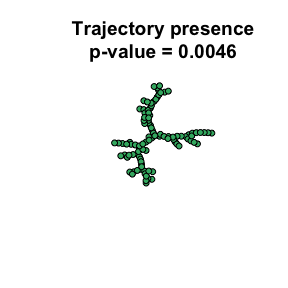pc=prcomp(input) plot(pc$x[,c(1:2)], xlab="PC1", ylab="PC2", cex=1,
sub="Principal components", col="mediumseagreen",
main = paste0("Trajectory presence\np-value = ",
format.pval(res$p.value, digit=2), "\n(n = ", nrow(input), ")") )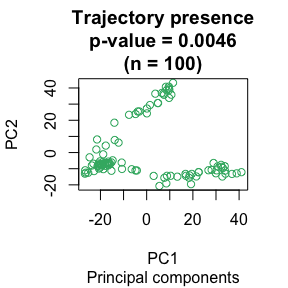Here we demonstrate using test.trajectory on the input matrix with modified parameter values. dim.reduction="pca" means dimensionality reduction will be performed first using principal component analysis. The number of principal components is selected using the Scree test. One can set dim.reduction="none" if dimensionality reduction is unnecessary. MST="exact"; the exact Euclidean MST (EMST) is used. The alternative is the approximate and fast dual-tree Boruvka algorithm by setting MST="boruvka" to obtain an approximate EMST. res = test.trajectory(input, dim.reduction = "pca", MST = "exact") Test results contained in res tdt_measure 2.6666667 statistic 74.8848818 tdt_effect 0.7488488 leaves 26.0000000 diameter 41.0000000 p.value 0.0046336 original_dimension 7848.0000000 pca_components 3.0000000 It can be seen from the results that the exact MST and boruvka MST are equivalent for small sample size. ### Example 1.2 Simulated isotropic data without trajectory presence We simulated spherical bivariate normal data, which are isotropic and contain no trajectory. The $$p$$-value for the test is insignificant. n = 100 mat = cbind(rnorm(n), rnorm(n)) res = test.trajectory(mat, dim.reduction = "none") Test statistics and $$p$$-value are contained in res: Test results contained in res tdt_measure 3.4242424 statistic 68.4820747 tdt_effect 0.6848207 leaves 27.0000000 diameter 32.0000000 p.value 0.8482481 We visualize the data which show no sign of trajectory presence. plot(res, node.size=12, main = paste0("Trajectory presence\np-value = ", format.pval(res$p.value, digit=2)))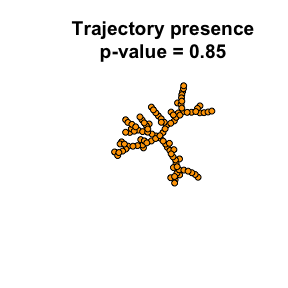plot(mat, col="orange", pch=2, cex=0.5, xlab="Dim 1", ylab="Dim 2",
main=paste0("Trajectory presence\np-value = ",
format.pval(res$p.value, digit=2), "\n(n = ", n, ")") )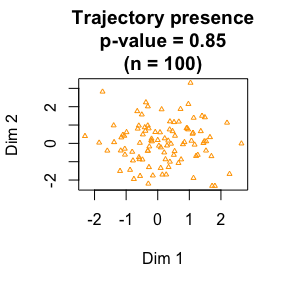### Calculating test statistics without $$p$$-value TDT statistics can be calculated relatively quickly to learn if there is any effect in the data before launching the more time consuming step of calculating the $$p$$-value. Function compute.stats() computes tree dimension measure, tree dimension effect, number of leafs and diameter of EMST for a given input data without calculating the $$p$$-value. res = compute.stats(mat, MST="boruvka", dim.reduction = "none") Results contained in res tdt_measure 3.4242424 tdt_effect 0.6848207 leaves 27.0000000 diameter 32.0000000 ## 2. Measuring data subset homogeneity by separability Function separability() computes heterogeneity of observations of the same type in a given data (Tenha and Song 2022). Observations of the same type have the same label. The function takes a matrix as input with rows as observations and columns as features. The function also takes a vector of labels for the observations. The function returns separability values for each label type and the overall separability value. The separability values range from 0 to 1, with 1 being the highest separability. ### Example 2.1 Homogeneous data with low separability In the examples below, there are three types of observations labeled L1, L2 and L3. An instance of real application is in single-cell data, where the labels could be cell types. #Random data mat = cbind(rnorm(200), rnorm(200)) #Labels for the samples in the data labels = c(rep("L1", 93), rep("L2",78), rep("L3",29)) #Color vector of samples, each unique color correspods with unique label cols = c(rep("orange", 93), rep("mediumseagreen", 78), rep("purple", 29)) #Compute separability of samples in mat res = separability(mat, labels) The result is a list containing separability values for each label and, overall separability on the data. The overall separability is relatively low, implying samples with same labels are mixed. knitr::kable(res$label_separability, col.names = "Label separability")
Label separability
L1 0.5535714
L2 0.4698795
L3 0.2710280
#Plots an MST of the data, with samples of the same label highlighted by same color
# plotTree(mat, labels, node.size = 12, node.col = cols,
#   main = paste("Low seperability", format(res$overall_separability, digits = 3)), # legend.cord=c(-2.1,0.9) # ) plot(res,node.size = 12, node.col = cols, main = paste("Low seperability", format(res$overall_separability, digits = 3)), legend.cord=c(-2.1,0.9))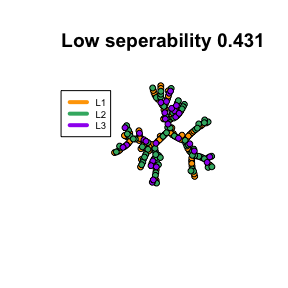### Example 2.2 Heterogeneous data with high separability

An example where samples with the same label are close together.

mat = rbind(
cbind(rnorm(93,mean=20), rnorm(93, mean=20)),
cbind(rnorm(78,mean=5), rnorm(78,mean=5)),
cbind(rnorm(29, mean=50), rnorm(29, mean=50)))
labels = c(rep("L1", 93), rep("L2",78), rep("L3",29))
res = separability(mat, labels)

The overall separability is 1, implying samples of different labels are perfectly separated.

knitr::kable(res$label_separability, col.names = " Label separability") Label separability L1 1 L2 1 L3 1 #Color vector of samples corresponding to labels cols = c(rep("orange", 93), rep("mediumseagreen", 78), rep("purple", 29)) # plotTree( # mat,labels, node.size=12, node.col = cols, # main = paste("High seperability", format(res$overall_separability, digits = 3)),
#   legend.cord=c(-1.9,0.9))

plot(res,node.size = 12, node.col = cols,
main = paste("High seperability", format(res$overall_separability, digits = 3)), legend.cord=c(-2.1,0.9))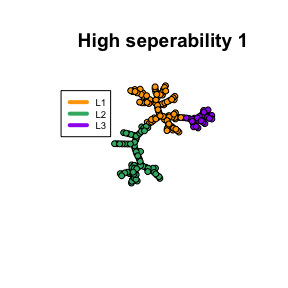## 3. Understanding tissue specificity of pathway gene expression dynamics via separability We now illustrate the use of separability to compute tissue specificity for calcium signaling and ribosome pathways on developing mouse data (Cardoso-Moreira et al. 2019) with samples of different tissue types. ### Example 3.1 Tissue specificity of the calcium signaling pathway during development #Loading calcium signaling pathway data from Mouse #This is Mouse development RNA-seq data spanned by the geneset fo calcium signaling pathway #Rows are genes and columns are samples. file.rdata=system.file('extdata', 'calcium_pathway_data.rdata', package = 'TreeDimensionTest') load(file=file.rdata) #loading color vector of samples by label type; mouse_cols file.rdata=system.file('extdata', 'mouse_cols.rdata', package = 'TreeDimensionTest') load(file=file.rdata) #Labels of the samples are the column names of the data, which are names of tissue types. labels = colnames(calcium_pathway_data) res = separability(t(calcium_pathway_data), labels) #Separabiltiy for each tissue type as well as the overall separability. High separability depicts high tissue specificity. # plotTree( # t(calcium_pathway_data), labels, node.col=mouse_cols,node.size=12, # main = paste("Calcium signaling pathway\nTissue specificity", # format(res$overall_separability, digits = 3)),
#   legend.cord=c(-1.9,-1.3))

plot(res,node.size = 12, node.col=mouse_cols,
main = paste("Calcium signaling pathway\nTissue specificity",
format(res$overall_separability, digits = 3)), legend.cord=c(-1.9,-1.3))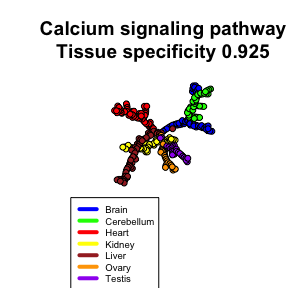Calcium signaling pathway has high tissue specificity as depicted by the high separability value. Tissues of the same type are closer together as shown in the plot. knitr::kable(res$label_separability, col.names = "Calcium signaling pathway tissue specificity")
Calcium signaling pathway tissue specificity
Brain 0.7971014
Cerebellum 1.0000000
Heart 1.0000000
Kidney 1.0000000
Liver 0.9047619
Ovary 0.9032258
Testis 0.8709677

### Example 3.2 Tissue specificity of the ribosome pathway during development

#Loading ribosome pathway data from Mouse
file.rdata=system.file('extdata', 'ribosome_pathway_data.rdata', package = 'TreeDimensionTest')

#loading color vector of samples by label type; mouse_cols
file.rdata=system.file('extdata', 'mouse_cols.rdata', package = 'TreeDimensionTest')

# ribsome_pathway_data is RNA-seq data with rows as genes and columns as samples
labels = colnames(ribosome_pathway_data)
res = separability(t(ribosome_pathway_data), labels)
plot(
res, node.col= mouse_cols,
node.size=12,
main = paste("Ribosome pathway\nTissue specificity",
format(res$overall_separability, digits = 3)), legend.cord=c(-1.9,-1.3))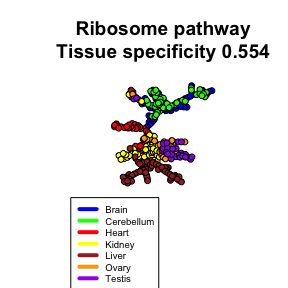The ribosome pathway has relatively lower tissue specificity as depicted by the lower separability value. Tissues of the same type are mixed as shown in the plot. knitr::kable(res$label_separability, col.names = "Ribosome pathway tissue specificity")
Ribosome pathway tissue specificity
Brain 0.5140187
Cerebellum 0.7818182
Heart 0.6179775
Kidney 0.6219512
Liver 0.5428571
Ovary 0.4057971
Testis 0.3913043